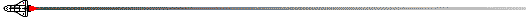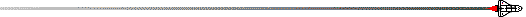## Making the Sun Shine by Nuclear FusionAll of these calculations are done in scientific notation to make it easier to write! Briefly, in scientific notation, the exponent of the 10 is equal to the number of places you move the decimal. For example, 1.0 x 105 is 100,000. Negatives work the same way but move the decimal in the opposite direction. So 1.0 x 10-5 is 0.00001.

The mass of a proton is 1.67 x 10-27 kg The reaction chain requires four protons as the mass input. A helium nucleus has a mass of 6.6326 x 10-27 kg and the 2 positrons produced during the reaction chain have a mass of 9.1139x10-31 kg each, making a total of 6.6344 x 10-27 kg total output mass. So after the reaction, there is less mass than there was before. Where did this mass deficit of 4.7227 x 10-29 kg go?  The most famous equation in the world (E=mc2) supplies the answer! How much energy will 4.7227 x 10-29 kg be converted into? (If m is in kg and c is in m/sec, E will be in units of Joules, J)

Now consider this:

We can measure the power output of the Sun to be L=3.9 x 1026 J/s. If each reaction in the proton-proton cycle produces 4.3 x 10-12 Joules, how many reactions must take place each second to power the Sun?

# reactions = 3.9 x 1026 / 4.3 x 10-12 = 9 x 1037

Remember we use up 4 protons in each reaction, so each second, we use up 3.6 x 1038 protons! How many protons are there in the Sun? We can get a quick estimate by calculating

# protons in the Sun ~ (total mass of Sun) / (mass of proton)

~ 2.0 x 1030 kg / 1.67 x 10-27 kg

~ 1.0 x 1057

If we have 1057 protons to use and we use 1038 each second, we have enough protons to last for about 1019 seconds…or over 100,000,000,000 years! It is important to note that the Sun will in fact use up only about 10% of its protons during its lifetime, however.The StarChild site is a service of the High Energy Astrophysics Science Archive Research Center (HEASARC), Dr. Alan Smale (Director), within the Astrophysics Science Division (ASD) at NASA/ GSFC.

StarChild Authors: The StarChild Team
StarChild Graphics & Music: Acknowledgments
StarChild Project Leader: Dr. Laura A. Whitlock
Curator: J.D. Myers
Responsible NASA Official: Phil Newman